# Two masons

Two masons built the garage together - it took 18 days. If they worked independently, the other would work 15 days more than the first. For how many days would build the garage each mason himhelp?

Correct result:

x =  30
y =  45

#### Solution:

$\dfrac1x + \dfrac 1y = \dfrac{1}{18} \ \\ y = x+15 \ \\ \ \\ \dfrac1x + \dfrac{1}{x+15} = \dfrac{1}{18} \ \\ \ \\ 18(2x+15) = x(x+15) \ \\ x^2 -21x -270 =0 \ \\ \ \\ a=1; b=-21; c=-270 \ \\ D = b^2 - 4ac = 21^2 - 4\cdot 1 \cdot (-270) = 1521 \ \\ D>0 \ \\ \ \\ x_{1,2} = \dfrac{ -b \pm \sqrt{ D } }{ 2a } = \dfrac{ 21 \pm \sqrt{ 1521 } }{ 2 } \ \\ x_{1,2} = \dfrac{ 21 \pm 39 }{ 2 } \ \\ x_{1,2} = 10.5 \pm 19.5 \ \\ x_{1} = 30 \ \\ x_{2} = -9 \ \\ \ \\ \text{ Factored form of the equation: } \ \\ (x -30) (x +9) = 0 \ \\ \ \\ x > 0 \ \\ x = 30$
$y=x+15=45$We would be pleased if you find an error in the word problem, spelling mistakes, or inaccuracies and send it to us. Thank you!Tips to related online calculators
Looking for help with calculating roots of a quadratic equation?
Do you have a linear equation or system of equations and looking for its solution? Or do you have quadratic equation?
Do you want to convert time units like minutes to seconds?

## Next similar math problems:

• DiscriminantDetermine the discriminant of the equation: ?
• EquationEquation ? has one root x1 = 8. Determine the coefficient b and the second root x2.
• Hectoliters of waterThe pool has a total of 126 hectoliters of water. The first pump draws 2.1 liters of water per second. A second pump pumps 3.5 liters of water per second. How long will it take both pumps to drain four-fifths of the water at the same time?Find the roots of the quadratic equation: 3x2-4x + (-4) = 0.
• Solve 3Solve quadratic equation: (6n+1) (4n-1) = 3n2
• RootsDetermine the quadratic equation absolute coefficient q, that the equation has a real double root and the root x calculate: ?
• Find the 20Find the product and the sum of the roots of x2 + 3x - 9 = 0
• Roots count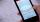Substitute the numbers/0,1,2,3/into the equation as x: (x - 1) (x - 3) (x + 1) = 0 Which of them is its solution? Is there another number that solves this equation?
• The product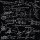The product of a number plus that number and its inverse is two and one-half. What is the inverse of this number
• Seven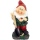Seven dwarfs will cut 420 stumps in 15 hours. After five hours, the two dwarves disappear discreetly. How many hours will the remaining dwarves complete the task?
• NovakNovak needed to dig up three of the same pit in the garden. The first pit dug father alone for 15 hours. His second dig son helped him and it did that in six hours. The third pit dug son himself. How long it took him?
• Invitations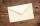For every 5 minutes writes Dana 10 invitations, while Anna 14 invitations. How long will write together 120 invitations?
• 2 pipes2 pipes can fill a tank in 35 minutes. The larger pipe alone can fill the tank in 24 minutes less time than the smaller pipe. How long does each pipie take to fill the tank alone?
• Wagons and cranesSeveral of the same cranes unloaded 96 wagons. If there were 2 more cranes there would be less 8 wagons for each crane. How many cranes were here?
• Three peopleThree people start doing a work at a same time. The first worked only 2 hours. The second ended 3 hours before the end. On an individual basis, it would take the first time to do the work 10 hours, second 12 hours and 15 hours third. How many hours did it
• Copiers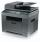The new copier copying a folder of papers 5 min. faster than the old. The operator used new, but out of toner and exchange took 5 min. In that time copied on the old. The whole work has been done for 9 min. How long would the work done only by old copier?
• Two pipes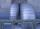One pipe fill one-fifth volume 20 minutes before by second one. The two pipes together will fill the tank in two hours. How long is will fill tank each pipe separately?